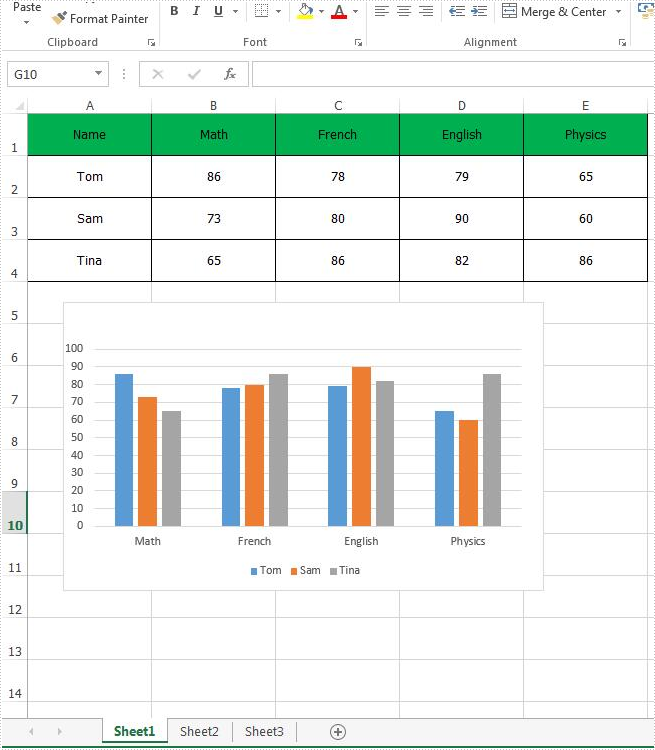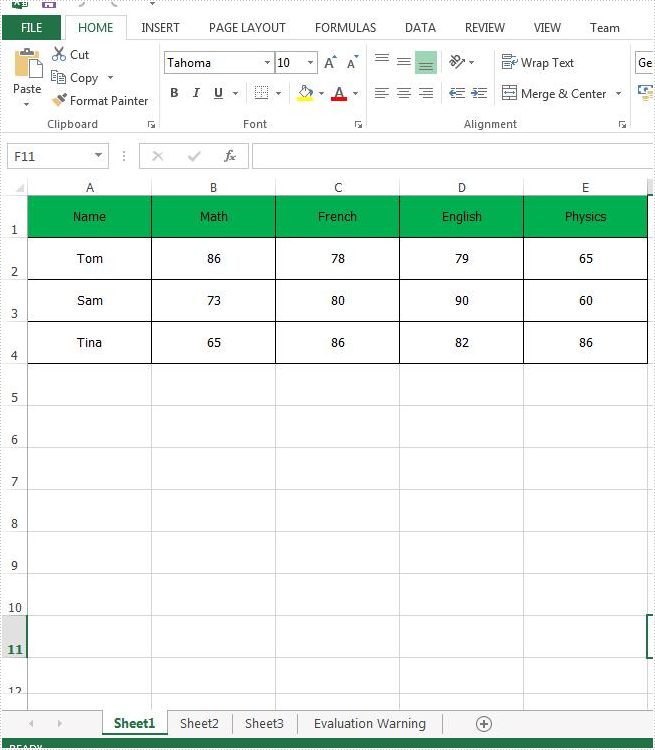# How to remove chart from Excel worksheet in C#, VB.NET

With Spire.XLS for .NET, we could easily add different kinds of charts to Excel worksheet, such as Pie Chart, Column Chart, bar chart, line chart, radar chart, Doughnut chart, pyramid chart, etc. In this article, we'll show you how to remove chart from Excel worksheet by using Spire.XLS.

Below is a sample document which contains a chart and table from the Excel worksheet, then we'll remove the chart from the slide.C# Code Snippet of how to remove the chart from Excel:

Step 1: Create an instance of Excel workbook and load the document from file.

```Workbook workbook = new Workbook();
```

Step 2: Get the first worksheet from the workbook.

```Worksheet sheet = workbook.Worksheets;
```

Step 3: Get the first chart from the first worksheet.

```IChartShape chart = sheet.Charts;
```

Step 4: Remove the chart.

```chart.Remove();
```

Step 5: Save the document to file.

```workbook.SaveToFile("RemoveChart.xlsx");
```

Effective screenshot:Full code:

[C#]
```using Spire.Xls;
using Spire.Xls.Core;
namespace RemoveChart
{

class Program
{

static void Main(string[] args)
{
Workbook workbook = new Workbook();
Worksheet sheet = workbook.Worksheets;
IChartShape chart = sheet.Charts;
chart.Remove();
workbook.SaveToFile("RemoveChart.xlsx");

}

}
}
```
[VB.NET]
```Imports Spire.Xls
Imports Spire.Xls.Core
Namespace RemoveChart

Class Program

Private Shared Sub Main(args As String())
Dim workbook As New Workbook()